Question

# In this phase diagram for water, indicate the direction that the solid-liquid and liquid-gas coexistence lines will m...

In this phase diagram for water, indicate the direction that the solid-liquid and liquid-gas coexistence lines will move after the addition of solute.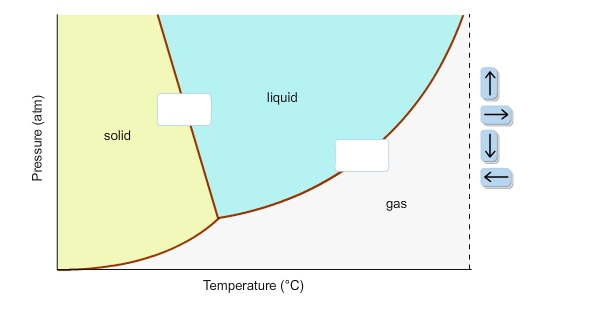In this phase diagram for water, indicate the direction that the solid-liquid and liquid-gas coexistence lines will move after the addition of solute.

Concepts and reason

The concept used to solve this problem is the phase diagram of water.

In the phase diagram of water, the solid-liquid coexistence line will move towards left after the addition of solute because of depression in freezing point of water upon the addition of solute.

The liquid-gas coexistence line will move towards right after the addition of solute because of elevation in boiling point of water upon the addition of solute.

Fundamentals

A phase diagram is a graph of pressure versus temperature depicting the solid, liquid and gaseous phases of a single substance under different conditions of temperature and pressure.

In the phase diagram of water, the solid-liquid coexistence line indicates the point at which the solid converts to liquid.

The temperature corresponding to $P = 1{\rm{ atm}}$ at the solid-liquid coexistence line in the phase diagram of the water is the freezing point of water.

When solute is added to the water, there is a lowering in the vapour pressure of the solution. Consequently, there is a lowering of freezing point of the water.

So, the direction of solid-liquid coexistence line will be towards the left, that is towards lower temperatures, after the addition of solute.

In the phase diagram of water, the liquid-gas coexistence line indicates the point at which the liquid converts to gas.

The temperature corresponding to $P = 1{\rm{ atm}}$ at the liquid-gas coexistence line in the phase diagram of the water is the boiling point of water.

When solute is added to the water, there is a lowering in the vapour pressure of the solution. Consequently, there is an elevation of boiling point of the water.

So, the direction of liquid-gas coexistence line will be towards the right, that is towards higher temperatures, after the addition of solute.

Ans:

The direction of solid-liquid and liquid-gas coexistence lines after the addition of solute is: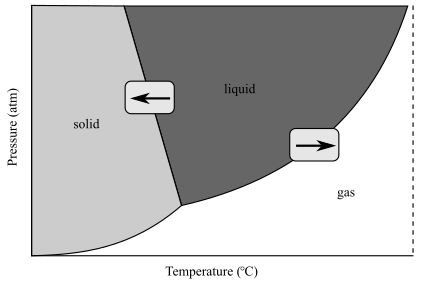#### Earn Coins

Coins can be redeemed for fabulous gifts.

Similar Homework Help Questions
• ### 7) Determine the slope in atm/K) of a solid-liquid coexistence line (in a P-T phase diagram)...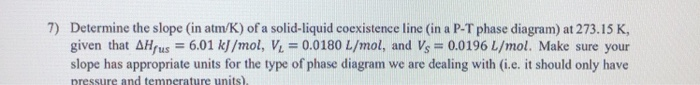7) Determine the slope in atm/K) of a solid-liquid coexistence line (in a P-T phase diagram) at 273.15 K, given that AHrus = 6.01 kJ/mol, V = 0.0180 L/mol, and Vs = 0.0196 L/mol. Make sure your slope has appropriate units for the type of phase diagram we are dealing with (i.e. it should only have pressure and temperature units)

• ### 9.2-1. In a particular solid-liquid phase transition the point Po, To lies on the coexistence curve....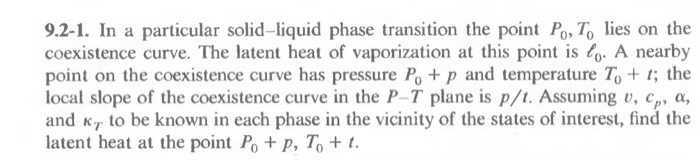9.2-1. In a particular solid-liquid phase transition the point Po, To lies on the coexistence curve. The latent heat of vaporization at this point is lo. A nearby point on the coexistence curve has pressure Po+ p and temperature To+ ; the local slope of the coexistence curve in the P-T plane is p/t. Assuming v, cp, a, and K to be known in each phase in the vicinity of the states of interest, find the latent heat at the...

• ### 71 Determine the slope (in atm/K) of a solid- liquid coexistence line (in a P-T phase...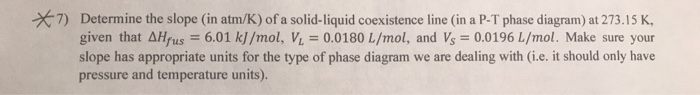71 Determine the slope (in atm/K) of a solid- liquid coexistence line (in a P-T phase diagram) at 273.15 K, given that AHfus = 6.01 kJ /mol, Vi 0.0180 L/mol, and Vs 0.0196 L/mol. Make sure your slope has appropriate units for the type of phase diagram we are dealing with (i.e. it should only have pressure and temperature units).

• ### Classify each phase as describing a solid, liquid, or gas. Solid Liquid Gas no definite volume,...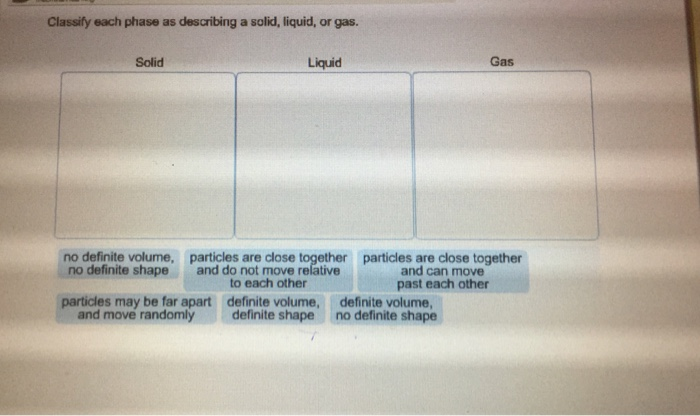Classify each phase as describing a solid, liquid, or gas. Solid Liquid Gas no definite volume, no definite shape particles are close together and do not move relative to each other particles are close together and can move past each other particles may be far apart and move randomly definite volume, definite shape definite volume no definite shape

• ### Phase changes are transitions between solid, liquid, and gas phases. For example, ice melting is an...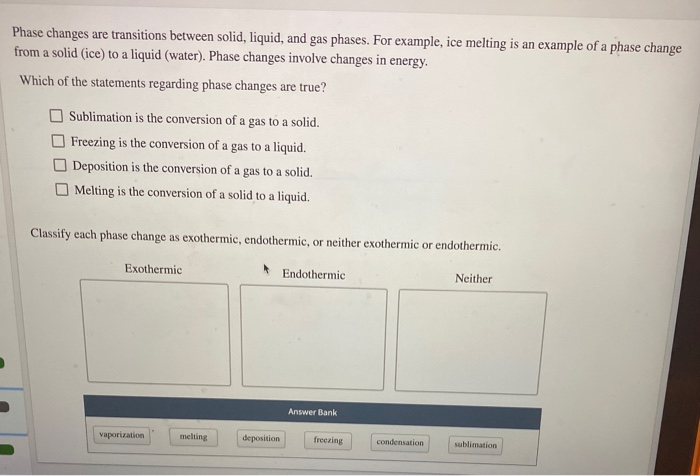Phase changes are transitions between solid, liquid, and gas phases. For example, ice melting is an example of a phase change from a solid (ice) to a liquid (water). Phase changes involve changes in energy. Which of the statements regarding phase changes are true? Sublimation is the conversion of a gas to a solid. Freezing is the conversion of a gas to a liquid. Deposition is the conversion of a gas to a solid. Melting is the conversion of a...

• ### GENERAL FEATURES OF A PHASE DIAGRAM; HYBRID ORBITALS AND FORMAL CHARGE MODEL 1 solid structure liquid...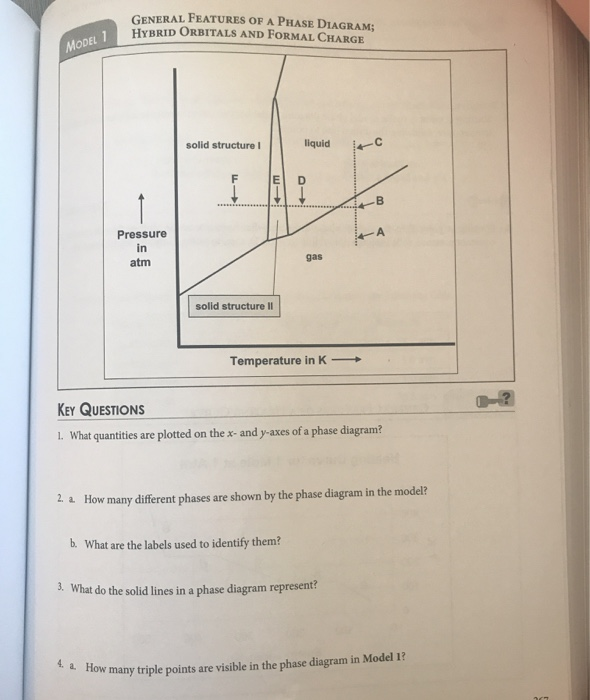GENERAL FEATURES OF A PHASE DIAGRAM; HYBRID ORBITALS AND FORMAL CHARGE MODEL 1 solid structure liquid rc Pressure in atm solid structure Il Temperature in K — KEY QUESTIONS 1. What quantities are plotted on the x-and y-axes of a phase diagram? 2. 2. How many different phases are shown by the phase diagram in the model? b. What are the labels used to identify them? 3. What do the solid lines in a phase diagram represent 4. 2. How...

• ### please help Study the following phase diagram of Substance X. 2.4 pressure (atm) solid liquid gas...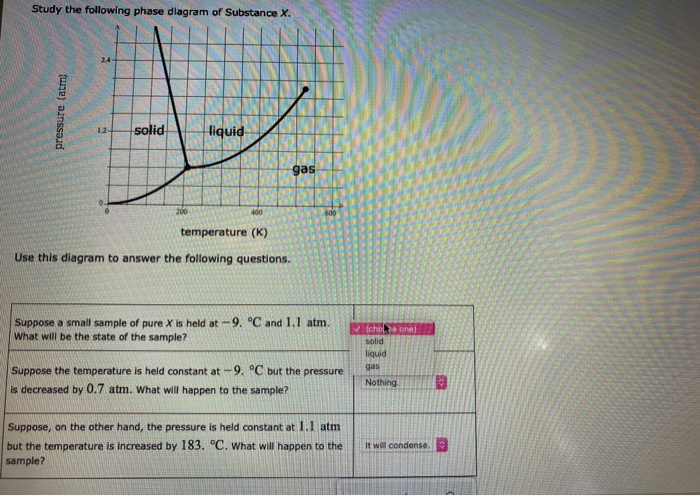please help Study the following phase diagram of Substance X. 2.4 pressure (atm) solid liquid gas 400 600 temperature (K) Use this diagram to answer the following questions. Suppose a small sample of pure X is held at -9. °C and 1.1 atm. What will be the state of the sample? ✓ (chole one) solid liquid gas Suppose the temperature is held constant at -9. °C but the pressure is decreased by 0.7 atm. What will happen to the sample?...

• ### Diagram 3 Solid Liquid Phase Diagram For Organics A And B 80 60 Temp °c) 40...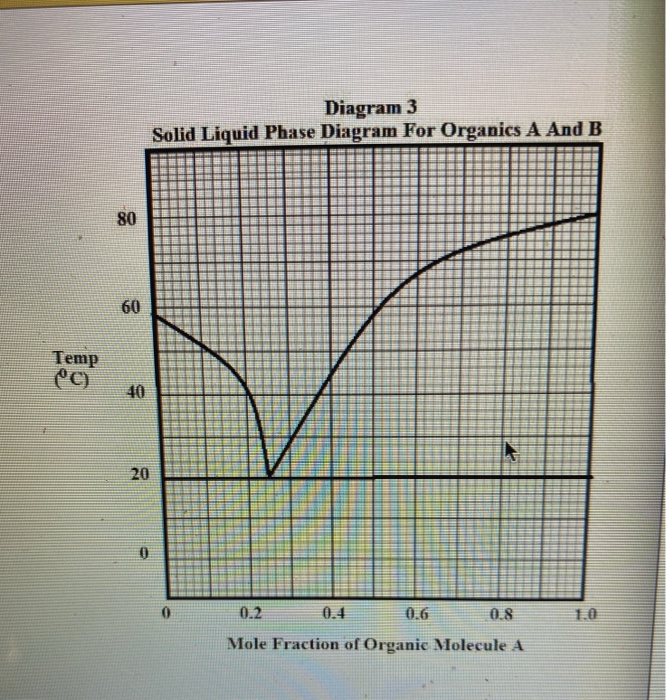Diagram 3 Solid Liquid Phase Diagram For Organics A And B 80 60 Temp °c) 40 20 0 0 0.2 0.6 0.8 1.0 Mole Fraction of Organic Molecule A Refer to the Diagram 3 The solid-liquid phase diagram for mixtures of Organic A (molar mass: 115.5 g/mole) and Organic B (molar mass = 98.4 g/mole). At what temperature does pure B melt? Ti = ? REPORT YOUR ANSWER TO 3 DIGITS ACCURACY DO NOT PUT UNITS IN THE ANSWER BOX...

• ### Study the following phase diagram of Substance X. solid pressure (atm) liquid gas 100 200 temperature...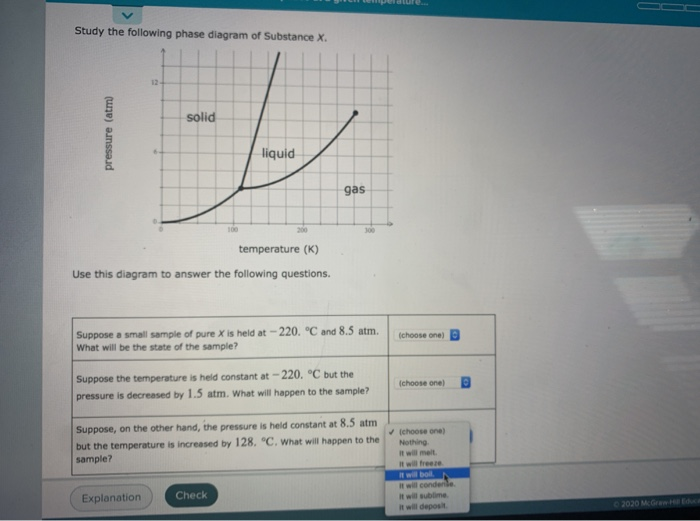Study the following phase diagram of Substance X. solid pressure (atm) liquid gas 100 200 temperature (K) Use this diagram to answer the following questions. Suppose a small sample of pure X is held at - 220 °C and 8.5 atm What will be the state of the sample? Ichoose one) choose one) Suppose the temperature is held constant at -220. °C but the pressure is decreased by 1.5 atm. What will happen to the sample? Suppose, on the other...

• ### 9. The phase diagram for Xenon is provided opposite Liquid What phase change(s) occur as Xenon...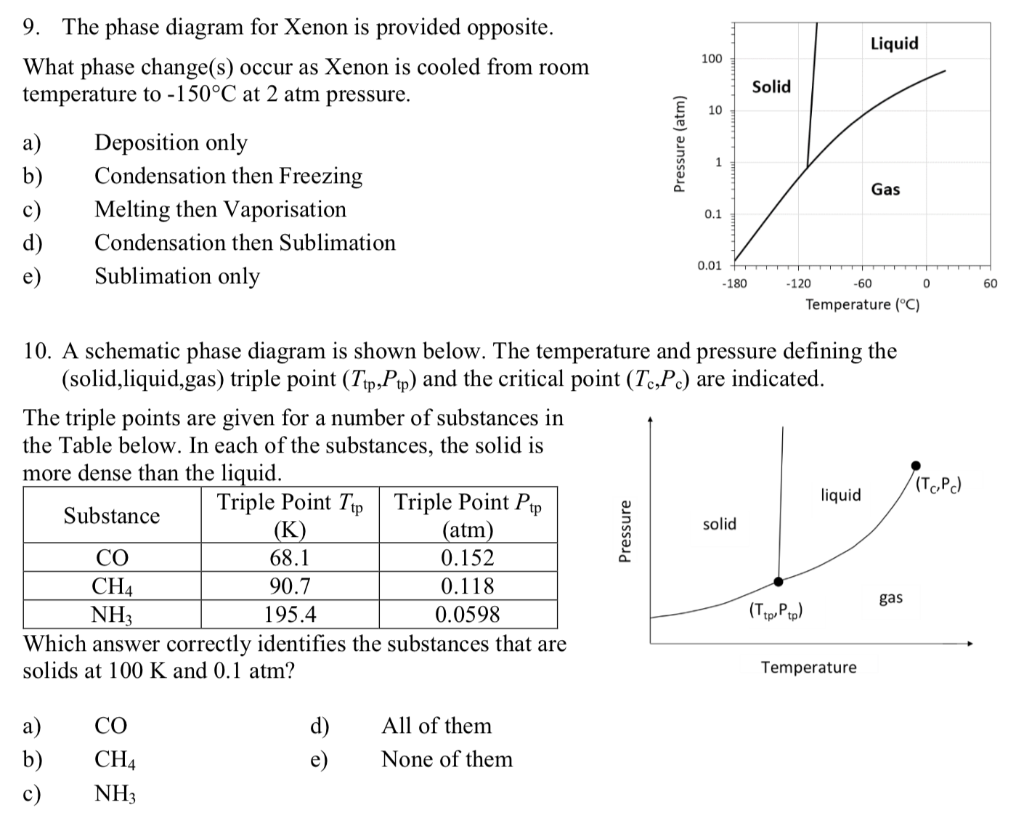9. The phase diagram for Xenon is provided opposite Liquid What phase change(s) occur as Xenon is cooled from room temperature to -150°C at 2 atm pressure 100 Solid 10 Deposition only Condensation then Freezing а) b) Gas c) Melting then Vaporisation 0.1 d) Condensation then Sublimation 0.01 Sublimation only е) -180 -120 -60 0 60 Temperature (C) 10. A schematic phase diagram is shown below. The temperature and pressure defining the (solid,liquid,gas) triple point (Tip,Pp) and the critical point...